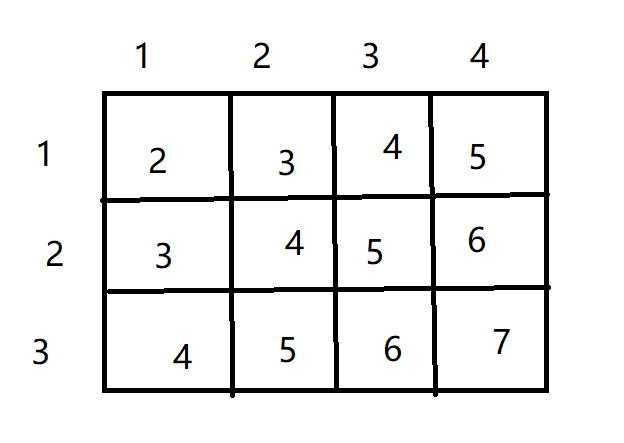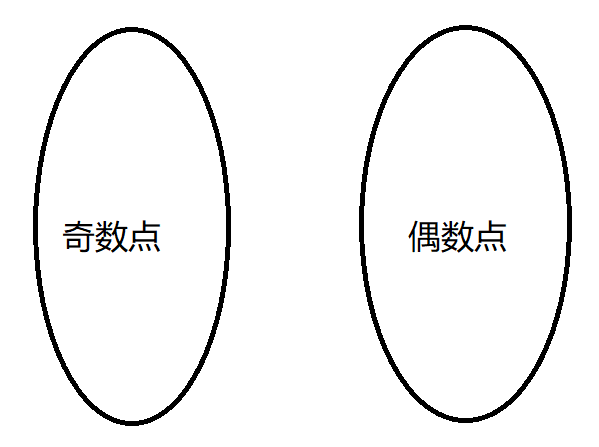# 棋盘覆盖

1 ≤ N ≤ 100

8 0

32

//

本题考查的是二分图匹配，因此我们可以考虑用匈牙利算法来解决这个问题
即然需要二分匹配，那么我们就得给这个图染个色才行，本题其实已经给我们染好色了~~
例：
我们将其横纵坐标加起来，就能发现其中的奥秘，**和为奇数点能与和为偶数的点匹配**，所就是说一个棋子一定覆盖一个和为偶数一个和为奇数的格子#include<iostream>
#include<cstring>
#include<queue>
#include<algorithm>
using namespace std;

const int N = 110;
pair<int,int>match[N][N]; //存储和为偶数点匹配的点
int n,t;
bool g[N][N],st[N][N];
int dx[] = {0,1,0,-1};
int dy[] = {1,0,-1,0};

int find(int x,int y) //匈牙利算法
{
for(int i = 0; i < 4; i++)
{
int xx = x + dx[i] , yy = y + dy[i]; // 遍历四周

if(st[xx][yy] || g[xx][yy])	continue; //该点已被访问过 或 不能放棋子

if( xx >= 1 && xx <= n && yy >= 1 && yy <=n ) //判断越界
{
st[xx][yy] = true; //表示该点已被访问过

int tx = match[xx][yy].first , ty = match[xx][yy].second;

if( (tx == 0 && ty == 0) || find(tx,ty))
{
match[xx][yy] = {x,y}; //匹配这两个点
return true;
}
}

}

return false;
}

int solve()
{
int res = 0;

for(int i = 1; i <= n; i++)
{
for(int j = 1; j <= n ;j++)
{
if(g[i][j])	continue;

if(( i + j ) % 2 == 0) //从奇数点开始匹配
{
memset(st,0,sizeof st);
if(find(i,j))	res++;
}
}
}

return res;
}

int main()
{
for(cin >> n >> t; t; t--)
{
int a,b;
cin >> a >> b;
g[a][b] = true; //标记不能放棋子的格子
}

cout << solve() << endl;

return 0;
}

10-10
12-1611-23
06-061万+
05-151808
03-17
09-24
11-10
05-11